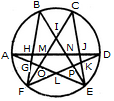# Non Verbal Reasoning - Analytical Reasoning - Discussion

Discussion Forum : Analytical Reasoning - Section 1 (Q.No. 3)
3.

Find the number of triangles in the given figure.22
24
26
28
Explanation:

The figure may be labelled as shown.The simplest triangles are AGH, GFO, LFO, DJK, EKP, PEL and IMN i.e. 7 in number.

The triangles having two components each are GFL, KEL, AMO, NDP, BHN, CMJ, NEJ and HFM i.e. 8 in number.

The triangles having three components each are IOE, IFP, BIF and CEI i.e. 4 in number.

The triangles having four components each are ANE and DMF i.e. 2 in number.

The triangles having five components each are FCK, BGE and ADL i.e. 3 in number.

The triangles having six components each are BPF, COE, DHF and AJE i.e. 4 in number.

Total number of triangles in the figure = 7 + 8 + 4 + 2 + 3 + 4 = 28.

Discussion:
29 comments Page 1 of 3.

Divya said:   3 years ago
The formula works: the total no. of nodes + 2*(no. of inner nodes).

16 + 2 * 6 = 28.

Inner Nodes means the nodes, not on the outline of the diagram.
(2)

Muthuraj said:   3 years ago
6+ (2*10) +2. Why do you add 2 in the last? Please explain.

Chandan said:   5 years ago
@Rahul.

How it is possible that Total number of nodes are lesser than inner nodes?

Chandan said:   5 years ago
@Rishikesh.

Can you explain how you got 16+2*4=28?

Rahul said:   7 years ago
Total no of nodes = 8,
inner nodes = 10,
= 8+2(10).
=28 ans.
(2)

Anoymous said:   7 years ago
How can we only consider I, L as inner nodes leaving J,K,G,H?

Avi Sharma said:   7 years ago
From the above figure indicated ay A, B, C, D, and so on..

TotalNumbers Of Nodes = 16 (A, B, C, D, E, F, G, H, I, J, K, L, M, N, O, P).
And Inner Nodes = 6 (M, N, O, P,I,L)

So Formula = Total no of Nodes + 2 * (Inner Nodes).
= 16 + 2 * 6,
(2)

JAISWAL said:   7 years ago
L is not inner node?

Neeraj batra said:   7 years ago
Thanks, @Lakshmikumar.

Rishikesh said:   7 years ago
From the above figure indicated ay A, B, C, D, and so on..

TTotalNumbers Of Nodes = 16 (A, B, C, D, E, F, G, H, I, J, K, L, M, N, O, P).
And Inner Nodes = 4 (M, N, O, P).

So Formula = Total no of Nodes + 2 * (Inner Nodes).
= 16 + 2 * 4.